# Augmented Matrix for a System of Equations

Matrices are a common tool used in algebra. They provide a way to deal with equations that have commonly held variables. In this post, we learn some of the basics of developing matrices.From Equation to Matrix

Using a matrix involves making sure that the same variables and constants are all in the same column in the matrix. This will allow you to do any elimination or substitution you may want to do in the future. Below is an example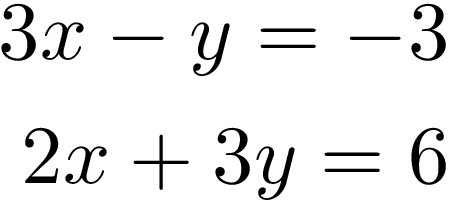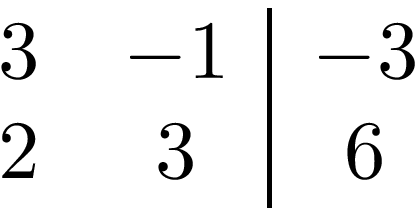Above we have a system of equations to the left and an augmented matrix to the right. If you look at the first column in the matrix it has the same values as the x variables in the system of equations (2 & 3). This is repeated for the y variable (-1 & 3) and the constant (-3 & 6).

The number of variables that can be included in a matrix is unlimited. Generally,  when learning algebra, you will commonly see 2 & 3 variable matrices. The example above is a 2 variable matrix below is a three-variable matrix.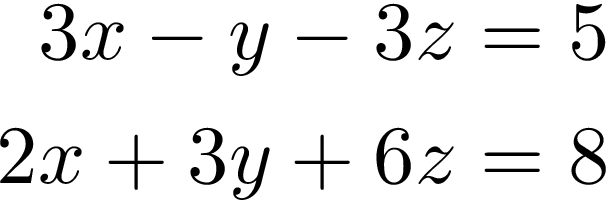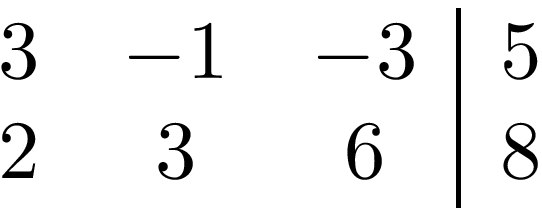If you look closely you can see there is nothing here new except the z variable with its own column in the matrix.

Row Operations

When a system of equations is in an augmented matrix we can perform calculations on the rows to achieve an answer. You can switch the order of rows as in the following.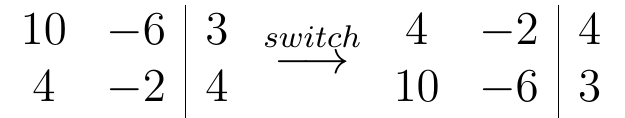You can multiply a row by a constant of your choice. Below we multiple all values in row 2 by 2. Notice the notation in the middle as it indicates the action performed.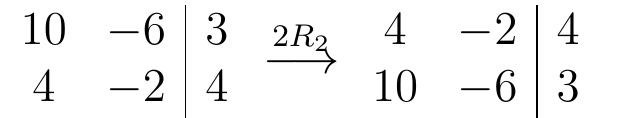You can also add rows together. In the example below row 1 and row 2, are summed to create a new row 1.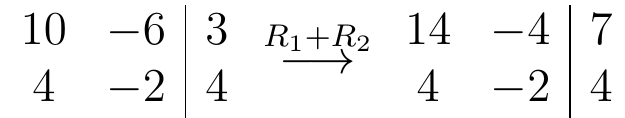You can even multiply a row by a constant and then sum it with another row to make a new row. Below we multiply row 2 by 2 and then sum it with row 1 to make a new row 1.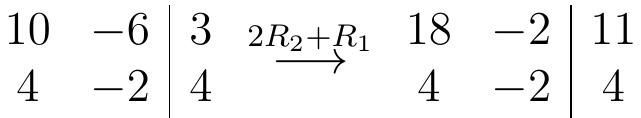The purpose of row operations is to provide a way to solve a system of equations in a matrix. In addition, writing out the matrices provides a way to track the work that was done. It is easy to get confused even the actual math is simple

Conclusion

System of equations can be difficult to solve. However, the use of matrices can reduce the computational load needed to solve them. You do need to be careful with how you modify the rows and columns and this is where the use of row operations can be beneficial.